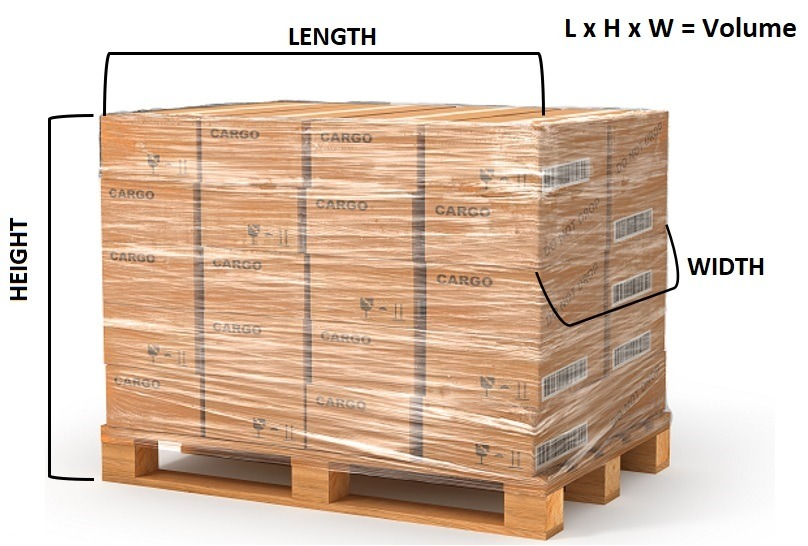Know the dimensions

### How To Measure Freight

To Measure the Cubic Volume of Freight
Cubing is the term given to measuring the size of a freight consignment
The Hunter Express Standard Cubic Conversion is 1 Cubic Metre = 250 Kilograms

(Over-sized freight of 1.2 metres or more will be charged as 333kg)

Example 1: Cubing a CartonWidth = 40 cm (or 0.4 of a metre)
Height = 35 cm (or 0.35 of a metre)
Length = 65 cm (or 0.65 of a metre)
Actual Weight = 15 kg

Multiply the height x length x width = m3 (cubic measurement)
= 0.35 x 0.65 x 0.4 = 0.091m3
To convert 0.091m3 to a weight in kilograms
Multiply 0.091m3 x 250 (standard cubic conversion) = 22.75 kg 22.75 kg is greater than the actual weight of 15 kg

Example 2: Cubing a PalletWidth = 1.2 m
Height = 1.9 m
Length = 1.2 m
Actual Weight = 450 kg

Multiply the height x length x width = m3 (cubic measurement) = 1.9 x 1.2 x 1.2 = 2.736m3
To convert 2.736m3 to a weight in kilograms
Multiply 2.736m3 x 250 (standard cubic conversion) = 684 kg
684 kg is greater than the actual weight of 450 kg

Example 3: Cubing an Odd Shaped ItemWidth = 1.4 m
Height = 1.6 m
Length = 1.3 m
Actual Weight = 580 kg
Multiply the height x length x width = m3 (cubic measurement) = 1.6 x 1.3 x 1.4 = 2.912m3

To convert 2.912m3 to a weight in kilograms
Multiply 2.912m3 x 250 (standard cubic conversion) = 728 kg
728 kg is greater than the actual weight of 580 kg